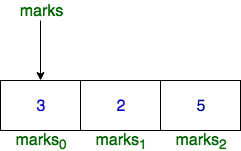# Students Marks Sum in C Hackerrank solution

You are given an array of integers, , denoting the marks scored by students in a class.

• The alternating elements  and so on denote the marks of boys.
• Similarly,  and so on denote the marks of girls.

The array name, , works as a pointer which stores the base address of that array. In other words,  contains the address where  is stored in the memory.

For example, let  and  stores 0x7fff9575c05f. Then, 0x7fff9575c05f is the memory address of .Function Description

Complete the function, marks_summation in the editor below.

marks_summation has the following parameters:

• int marks[number_of_students]: the marks for each student
• int number_of_students: the size of marks[]
• char gender: either 'g' or 'b'

Returns

• int: the sum of marks for boys if , or of marks of girls if

Input Format

• The first line contains , denoting the number of students in the class, hence the number of elements in .
• Each of the  subsequent lines contains .
• The next line contains .

Constraints

•  (where )
•  =  or

Sample Input 0

3
3
2
5
b
Sample Output 0
8Explanation 0Explanation 0 = [3, 2, 5] and  = .So, .Sample Input 1
5
1
2
3
4
5
g
Sample Output 1
6Explanation 1 = [1, 2, 3, 4, 5] and  = So,  =  = .Sample Input 2
0Explanation 2 =  and  = Here,  does not exist. So,  = .
#include <stdio.h>
#include <string.h>
#include <math.h>
#include <stdlib.h>

int marks_summation(int* marks, int number_of_students, char gender)
{
int sum = 0;

if(gender == 'b'){
for(int i = 0; i < number_of_students; i += 2)
sum += marks[i];
}
else{
for(int i = 1; i < number_of_students; i += 2)
sum += marks[i];
}

return sum;
}

int main()
{
int number_of_students;
char gender;
int sum;

scanf("%d", &number_of_students);
int *marks = (int *) malloc(number_of_students * sizeof (int));

for (int student = 0; student < number_of_students; student++) {
scanf("%d", (marks + student));
}

scanf(" %c", &gender);
sum = marks_summation(marks, number_of_students, gender);
printf("%d", sum);
free(marks);

return 0;
}
Getting Info...